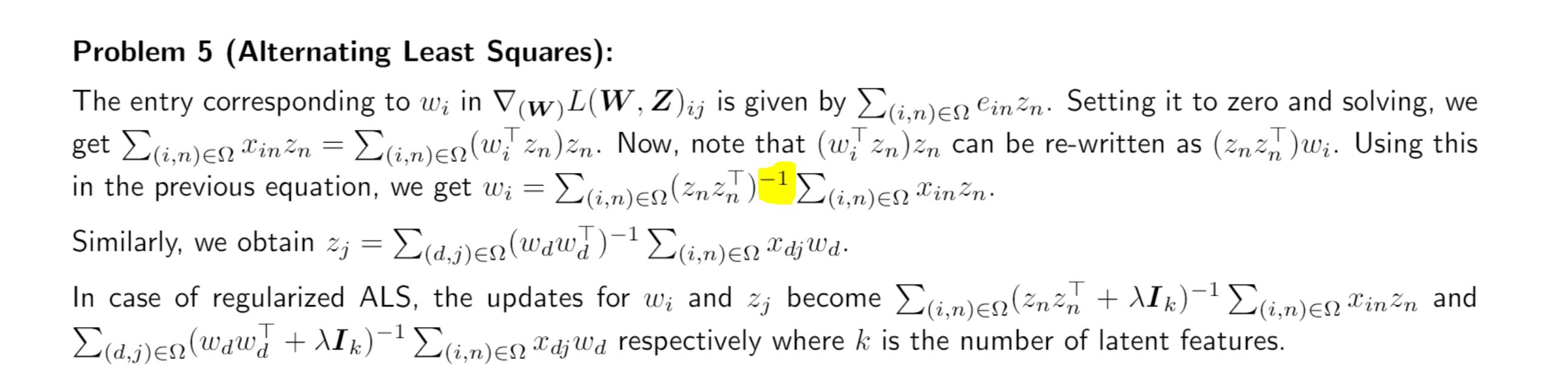### ALS with missing entries

Hi,

I wanted to confirm the inverse is taken after the sum right? it's not a sum of inverses?

Also I am not sure how do we compute complexity for such a step?

ThanksHello,
The corrected solution has been uploaded. Taking inverse after the sum is correct indeed

thank you! and how would should we go about calculating the complexity?

Each step(updating W or Z) is similar to computing solution for a similar Linear Regression(Ridge in case of regularized)

I have a question about the sign of the gradients: shouldn't we have $$dL/dw_i = - \Sigma z_n(x_{in}-(WZ^T)_{in})$$ for the first one for example?? :'(

Also, since $$w_i$$ and $$z_n$$ are rows, the dimensions of $$w_i^T z_n$$ are not correct for every entry of $$(WZ^T)_{in}$$, it should be $$w_i z_n^T$$, shouldn't it ? What do you guys think ?

Yes that actually makes sense after checking the dimensions of the final result (for example, the inverse was hinting at the fact that the product resulted in a matrix, not a scalar). What about the minus sign ? Of course, I know it doesn't change the result, but just for rigour

Page 1 of 1